# Day 1 Assignment (Series)

I am back once again with lots of Salesforce programming practice questions for beginners. I have created a 5 Days Salesforce based programming and configuration assignments for practice and improving your skills. This blog have the first day assignment of creating various series using Salesforce Apex language. Writing down scripts on Execute anonymous we can produce the following series. New developers with little or no knowledge of programming can start their practice from these questions.

#### Output like this (1 , 4 , 8 , 32 , 64 , 256 , 512 , …..);  For Loop in Salesforce.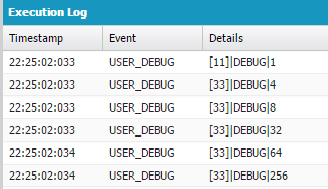public with sharing class Series

{

public integer display(integer userInput)

{

integer i = 1;

system.debug(i);

for(integer a=1 ; a<=userInput ; a++)

{

if(math.mod(a,2)==0)

{

i=i*2;

}

else

{

i=i*4;

}

system.debug(i);

}

return i;

}

}

####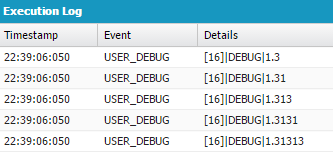public with sharing class Series2 {

public Series2(integer num)

{

double d = 1.0;

for(integer i =1;i<=num;i++)

{

if(math.mod(i,2)==0)

{

d=d+math.pow(10,-i);

}

else

{

d=d+(3*(math.pow(10,-i)));

}

system.debug(d);

}

}

}

3.Print all the Factors of a number. Example input: 84

Output: Factors (2 , 3 , 4 , 6, 7, 12,14 , 21,28, 42);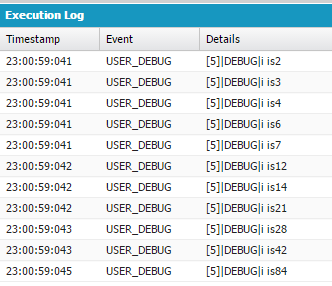public with sharing class Series3

{

public Series3(integer num)

{

for(integer i =2;i<=num;i++)

{

if(math.mod(num,i)==0)

{

system.debug(i);

} } } }

4.Print a Series which produce

Output like this(522 , 502 , 492 , 472 , 462 , 442 , …);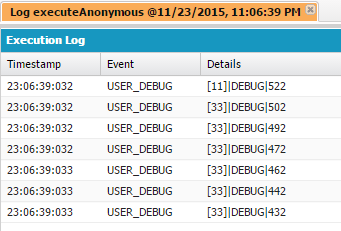public with sharing class Series5

{

public integer display(integer userinput)

{

integer i = 522;

system.debug(i);

for(integer a=1 ; a<=userInput ; a++)

{

if(math.mod(a,2)==0)

{

i=i-10;

}

else

{

i=i-20;

}

system.debug(i);

}

return i;

} }

5. Take any number and print its Units, Tens, Hundreds, Thousand etc. Example input: 293,023

Output like this : 200,000, 90,000, 3,000, 0, 20, 3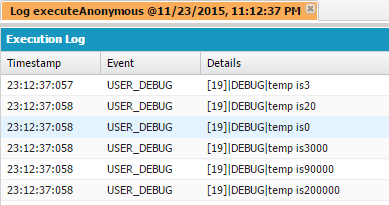public with sharing class Units

{

public void show(integer n)

{

integer val=1;

while(n>0){

integer temp=math.mod(n,10);

n=n/10;

temp=temp*val;

system.debug(‘temp is’+temp);

val=val*10;

}

}

}

6. Take any number and print its Units, Tens, hundreds, thousand etc both in decimal and integer part. Example input: 293.065.

Output: 200.0, 90.0, 3.0, 0.0, 0.06, 0 .005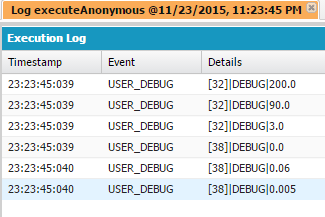public with sharing class UnitPlacesOfDecimalNumber {

public void showNumbers(Decimal originalNumber) {

Decimal num = originalNumber;

//To Seperate Integer Part and Decimal Part of the number.(Like 293.065 -> 293 and .065)

Integer intPartOfNumber = (Integer) num;

Decimal decPartOfNumber = num – intPartOfNumber;

List<Integer> intNumbersList = new List<Integer>();

//To get List of Integer Part.(Like 293 -> 3, 9, 2)

Integer n = 1;

while(intPartOfNumber > 0) {

n = math.mod(intPartOfNumber, 10);

intPartOfNumber = intPartOfNumber/10;

}

//To get List of Decimal Parts.(Like .065 -> 0, 6, 5)

List<Integer> decimalNumberList = new List<Integer>();

Decimal decPart = 1;

while(decPartOfNumber > 0) {

decPart = decPartOfNumber * 10;

intPartOfNumber = (Integer) decPart;

decPartOfNumber = decPart – intPartOfNumber;

}

//To Print Int Part of the number with unit places.(Like 293 -> 200, 90, 3)

for(Integer i = intNumbersList.size(); i >= 1; i–) {

System.debug((Integer) intNumbersList.get(i-1) * math.pow(10, i-1));

}

}

}Author: AJ

1.Hi AJ,

I am new to Apex classes.

If I execute the program in Execute Anonymous.. It didnt display any output.

Could you help me out. How should I execute this programs in Execute Anonymous

•I got it AJ. I tried, Now I understand how to call a method from Execute Anonymous.

2.Hi – I am trying the first assignment, I find it difficult to execute it. I executed as below :
Series.display(10); but it is throwing the following error

Non static method cannot be referenced from a static context: Integer Series.display(Integer)

Kindly let me know if I am executing something wrong.

Thanks!

•